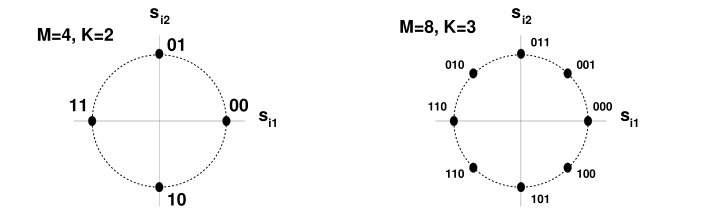# phase shift keying

Modulation scheme encoding all information into phase.

\begin{equation} s_i(t) = \Re\{Ag(t)e^{j2\pi (i-1)/M} e^{j2\pi f_c t}\} \end{equation}

signal constellation points are given by

\begin{equation} s_i1 = A \cos \left(\frac{2\pi (i-1)}{M} \right) \end{equation}
\begin{equation} s_i2 = A \sin \left(\frac{2\pi (i-1)}{M} \right) \end{equation}

All signals have equal energy, $$A^2$$.

Based on the number of points in the diagram, $$M$$ (because we need to map $$M$$ messages onto coefficients in signal space), there are a few popular variants of PSK. Namely

2PSK
binary phase shift keying
4PSK